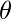# Exercise: Questions on Array

Questions on Array : Question 1 :
A program P reads in 500 integers in the range [0..100] exepresenting the scores of 500 students. It then prints the frequency of each score above 50. What would be the best way for P to store the frequencies? (GATE CS 2005)

 An array of 50 numbers  Correct An array of 100 numbers An array of 500 numbers A dynamically allocated array of 550 numbers
Questions on Array : Question 2 :
Which of the following operations is not O(1) for an array of sorted data. You may assume that array elements are distinct.

 Find the ith largest element Delete an element  Correct Find the ith smallest element All of the above
Questions on Array : Question 3 :
The minimum number of comparisons required to determine if an integer appears more than n/2 times in a sorted array of n integers is
(A)(n)
(B)(logn)
(C)(log*n)
(D)(1)

 A B  Correct C D
Questions on Array : Question 4 :
Let A be a square matrix of size n x n. Consider the following program. What is the expected output?

C = 100
for i = 1 to n do
for j = 1 to n do
{
Temp = A[i][j] + C
A[i][j] = A[j][i]
A[j][i] = Temp - C
}
for i = 1 to n do
for j = 1 to n do
Output(A[i][j]);


 The matrix A itself  Correct Transpose of matrix A Adding 100 to the upper diagonal elements and subtracting 100 from diagonal elements of A None of the above